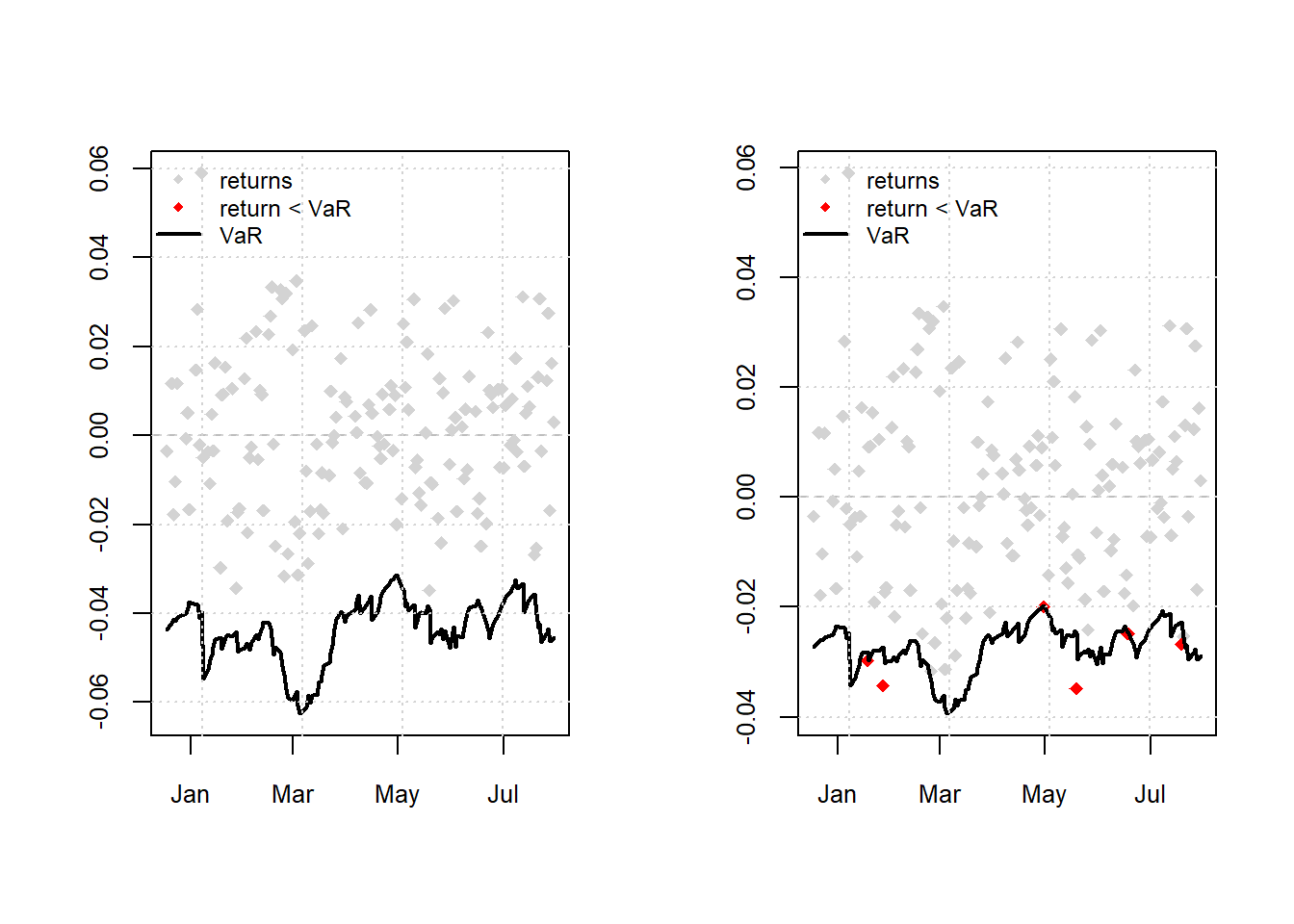## 11.4 VaR forecasts using out of sample

• Let’s use Student-t distribution as Financial Returns don’t always follow normal distribution
# spec2 with student-t distribution
spec2 = ugarchspec(variance.model = list(model = "sGARCH", garchOrder = c(1,
1)), mean.model = list(armaOrder = c(0, 0)), distribution.model = "std")
• The rugarch package has a very useful function for estimating moving window models and forecasting VaR. The function provides method for creating rolling forecasts from GARCH models and has various arguments to specify the forecast length (), window size (), model refitting frequency (), a rolling or recursive estimation window () etc.
var.t = ugarchroll(spec2, data = bhp_ret, n.ahead = 1, forecast.length = ndays(bhp_ret) -
500, refit.every = 5, window.size = 500, refit.window = "rolling",
calculate.VaR = TRUE, VaR.alpha = c(0.01, 0.05))
• We can plot the 1% and 5% VaR forecasts against the actual returns using the following routine.
# note the plot method provides four plots with option-4 for the VaR
# forecasts 1% Student-t GARCH VaR
par(mfrow = c(1, 2))
plot(var.t, which = 4, VaR.alpha = 0.01)
# 5% Student-t GARCH VaR
plot(var.t, which = 4, VaR.alpha = 0.05)Figure 11.3: Actual Return Vs 1% VaR forecasts

• Finally backtesting can be obtained using the report method
# backtest for VaR forecasts

report(var.t, VaR.alpha = 0.05)  #default value of alpha is 0.01
VaR Backtest Report
===========================================
Model:              sGARCH-std
Backtest Length:    154
Data:

==========================================
alpha:              5%
Expected Exceed:    7.7
Actual VaR Exceed:  6
Actual %:           3.9%

Unconditional Coverage (Kupiec)
Null-Hypothesis:    Correct Exceedances
LR.uc Statistic:    0.426
LR.uc Critical:     3.841
LR.uc p-value:      0.514
Reject Null:        NO

Conditional Coverage (Christoffersen)
Null-Hypothesis:    Correct Exceedances and
Independence of Failures
LR.cc Statistic:    0.916
LR.cc Critical:     5.991
LR.cc p-value:      0.633
Reject Null:        NO